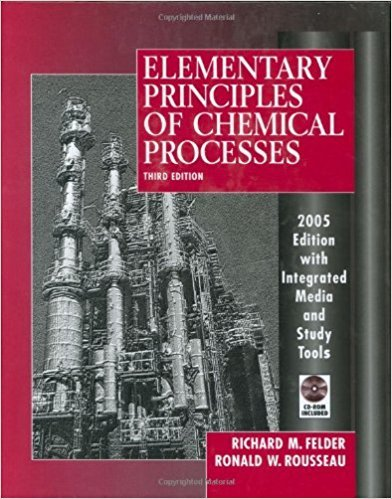×
Get Full Access to Elementary Principles Of Chemical Processes - 3 Edition - Chapter 11 - Problem 11.5
Get Full Access to Elementary Principles Of Chemical Processes - 3 Edition - Chapter 11 - Problem 11.5

×

# A gas storage tank with a floating roof receives a steadyISBN: 9780471687573 143

## Solution for problem 11.5 Chapter 11

Elementary Principles of Chemical Processes | 3rd Edition

• Textbook Solutions
• 2901 Step-by-step solutions solved by professors and subject experts
• Get 24/7 help from StudySoup virtual teaching assistantsElementary Principles of Chemical Processes | 3rd Edition

4 5 1 278 Reviews
27
2
Problem 11.5

A gas storage tank with a floating roof receives a steady input of 540 m3 /h of a natural gas. The rate of withdrawal of gas from the tank. vw , varies more or less randomly during the day and is recorded at lO-min intervals. At 8:00 a.m. one morning the volume of stored gas is 3.00 x 103 m3 . The withdrawal rate data for the next 4 hours are as follows: Hour Beginning at 8:00 9:00 10:00 11:00 12:00 11.4,11.9.12.1,11.8,11.5,11.3 11.4, 11.1, 10.6, 10.8, 10.4, 10.2 10.2, 9.8, 9.4, 9.5, 9.3, 9.4 9.5, 9.3, 9.6, 9.6, 9.4, 9.9 9.8 Student Workbook Encvclopedia Equipment valve The temperature and pressure of the inlet, stored, and outlet gases are equal and nearly constant throughout the given time period. (a) Write a differential balance on the moles of gas in the tank, and prove that when integrated it yields the following equation for the gas volume: Vet) = 3.00 X 103 + 9.00t - J: Vw dt where t(min) is the time elapsed since 8:00 a.m. (b) Calculate the stored gas volume at noon, using Simpson's rule (Appendix A.3) to evaluate the integral. (c) Although a running estimate of the tank volume is important to have, in practice it would probably not be obtained in the manner indicated. How would it be obtained? What might you infer if the value estimated in part (b) is greater than that obtained by the more accurate method? *(d) Create a spreadsheet or a computer program to read in the data for vw(t) and to estimate and print out the gas volume at each time for which Vw is recorded, using the trapezoidal rule (Appendix A.3) to evaluate the integral over each successive lO-min interval. What is the percentage difference between the volumes at noon estimated by Simpson's rule and the trapezoidal rule? Which estimate should be more accurate?

Step-by-Step Solution:
Step 1 of 3

CHM 1045 WEEK 8 NOTES Gas pressure is the result of the force of gas molecules when colliding with the surfaces of objects ­ 760 mm Hg = 760 torr = 1 atm = 101325 Pa Gay­Lussac’s law. ­ the pressure is directly related to temperature with constant volume ­ When temperature increase, pressure increase. ­ Temperature must be in Kelvin ­ P1/T1=P2/T2 Charles’s law ­ Volume is directly related to temperature with constant temperature. ­ Volume increases as the temperature increases, ­ Temperature must be in Kelvin ­ v1/t1=v2/t2 Boyle’s law ­ Volume is inversely related to pressure with constant temperature ­ When pressure increase, volume decrease ­ p1v1=p2v2 Avogadro’s law ­ Mole of a gas is directly related to its volume with constant p

Step 2 of 3

Step 3 of 3

##### ISBN: 9780471687573

Unlock Textbook Solution